# Will ChatGPT Replace Programmers At LEDAS?

Nikita Vorobyov

You must have heard of ChatGPT, that artificial intelligence chatbot developed by OpenAI. It interacts with humans in a conversational way by supporting queries made in natural languages.

For developers, ChatGPT’s most attractive feature is its ability to generate code in any programming language. But how useful is AI-generated code? Let's find out by asking ChatGPT to solve some simple tasks that arise in CAD development projects. (We conducted this study some time ago; since then, ChatGPT has perhaps become smarter.)

1. From scratch, write a geometric algorithm to find the intersection of two lines in 3D space
2. Using an open source library, write a geometric algorithm to imprint a 3D solid
3. Provide a brief guide to developers on how to implement an algorithm that offsets solids

The first two had to be coded in C++; the third is a description.Image created by AI

### Test 1. Intersection of Two Lines Algorithm

##### Task 1a: Write the algorithm in C++

We began by asking ChatGPT to write an algorithm that finds the intersection point of two lines in three-dimensional space. We wrote our request like this:

“For the following task use C++ code.
Create structures describing 3D points, vectors and lines.
Write a function which finds the intersection point between two 3D lines.”

In response, the chatbot generated the following code for function findIntersection:It also provided a few tests with sample data. Here is one of them: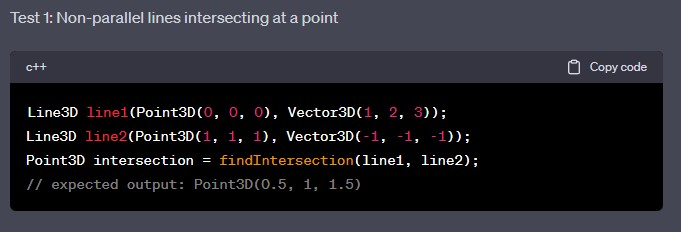This code compiles, but the algorithm did not work, as it calculated the problem incorrectly.

ChatGPT apparently decided to use Cramer's rule for the solution of systems of equations, but was confused by the coefficients. Looking at the calculation of the determinant of the matrix (the denom variable) and the x and y coordinates, we can see that it made a mashup of algorithms for 2D lines and 3D ones.

We next asked ChatGPT to explain its code, but after several attempts it could only write something similar to comments in programming code, and did not explain the idea behind the approach it used. Here is its explanation:

“This code finds the intersection point of two 3D lines by solving a system of equations. In this part of the code, the coefficients for calculating the parameters t1 and t2 are calculated.
– First, the denominator denom is calculated as follows: denom = a1 * b2 - a2 * b1, where a1, b1 and a2, b2 are the components of the direction vectors of the first and second line, respectively.
– If the denominator is not equal to 0, then the values of the parameters t1 and t2 are calculated using the formulas…”

And so on. When we asked it to fix the code, ChatGPT offered this alternative solution: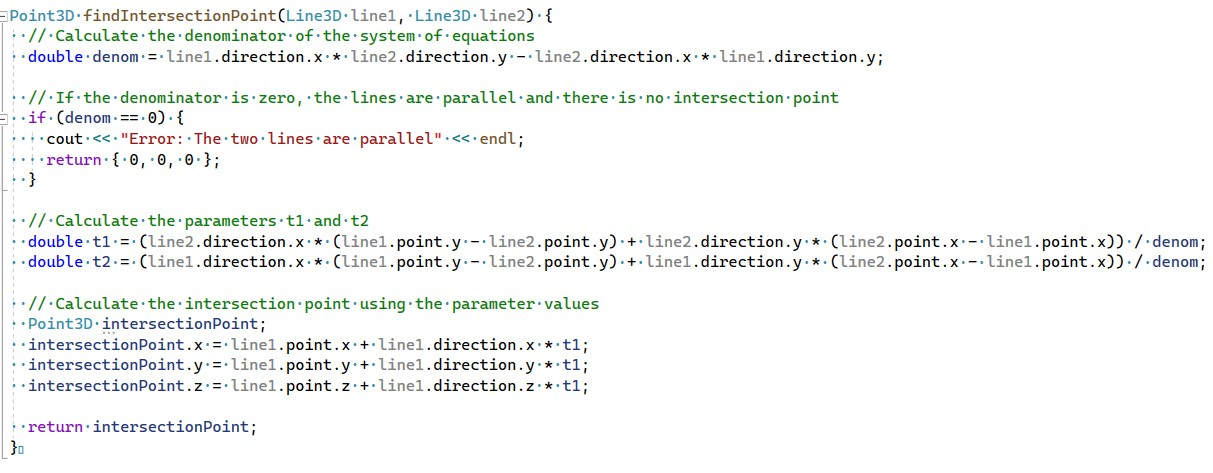However, it failed again. Our attempts to ask the chatbot about the problems also failed: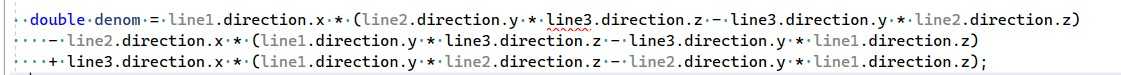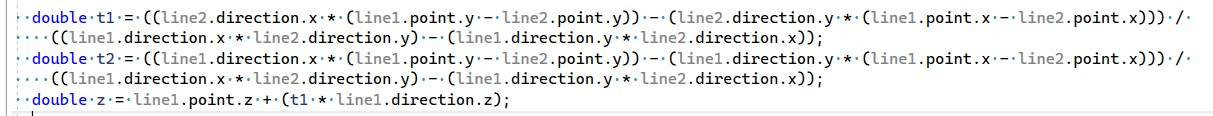It appears that it was difficult for the chatbot to operate with similarly-named variables like t1 and line1. For example, in the images above, you can see reference to a line3 variable, even though only two lines are being intersected.

With each new attempt to fix the generated code, more and more errors appeared, and eventually the code no longer compiled. After ChatGPT generated the code shown below, we decided to stop torturing it with this task.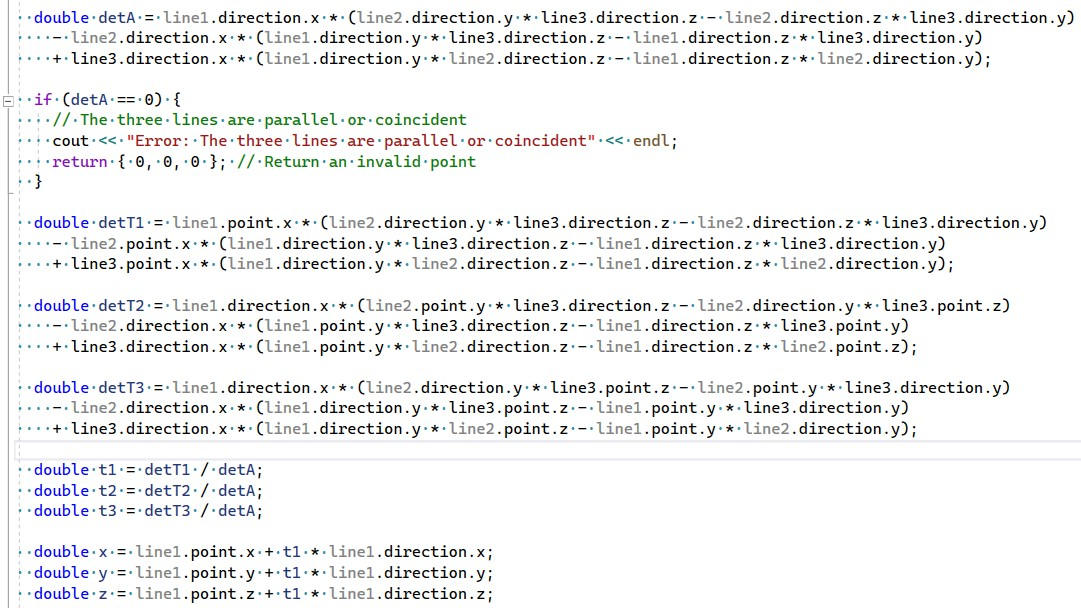##### Task 1b: Write the algorithm in Python

We decided that ChatGPT perhaps could not complete the first task due to the complexity of the C++ programming language, so we asked it to code the algorithm in Python instead. The first version of the new code did not work, because ChatGPT was trying to use a NumPy library that wasn't imported:When we let ChatGPT use NumPy, the code worked!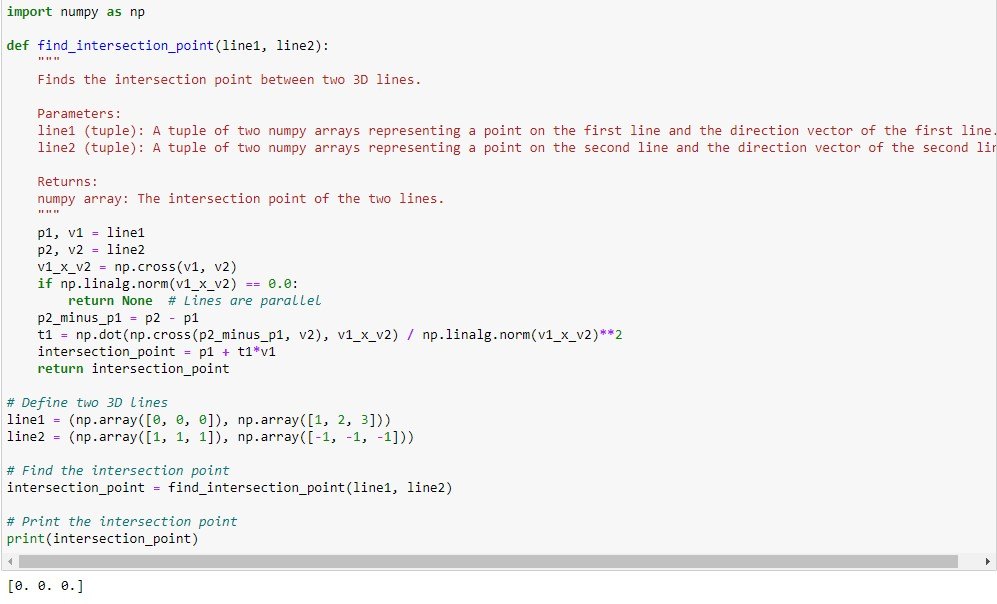The algorithm passed all tests when we submitted different input data.

Summary

• ChatGPT was not able to write valid C++ code for the algorithm, but suggested an idea for solving the task and generated basic code that can be modified further by humans.
• ChatGPT was better at generating Python code, perhaps because the syntax of this programming language is simple, or because there may well be many more Python examples on which it was trained.

Our next test for ChatGPT was to implement a new simple algorithm for the OpenCASCADE geometric kernel. We chose the Imprint function, because it is not yet implemented in OpenCASCADE and is quite simple. In geometric kernels, the Imprint operation "prints" one body onto another, removing the resulting intersection body from the second body.

The algorithm carries out the following steps:

1. Intersect two input solids
2. Find all boundary elements of the intersection (curves and points)
3. Subdivide the faces of one of the solids with these curves and points to create a new topology, and then generate a new solid body

For example, the image below shows result of imprinting the red solid on the blue one. The result has a new set of edges on the front face of the blue cube.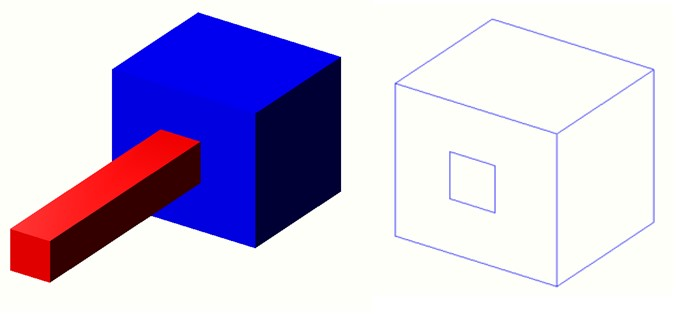For our first request, we instructed ChatGPT to use specific tools to implement the task, briefly explaining the function of Imprint: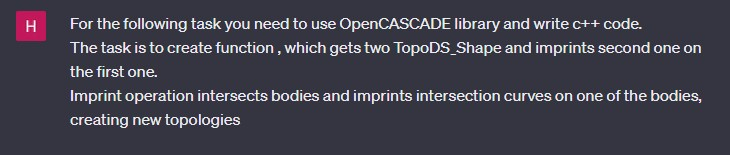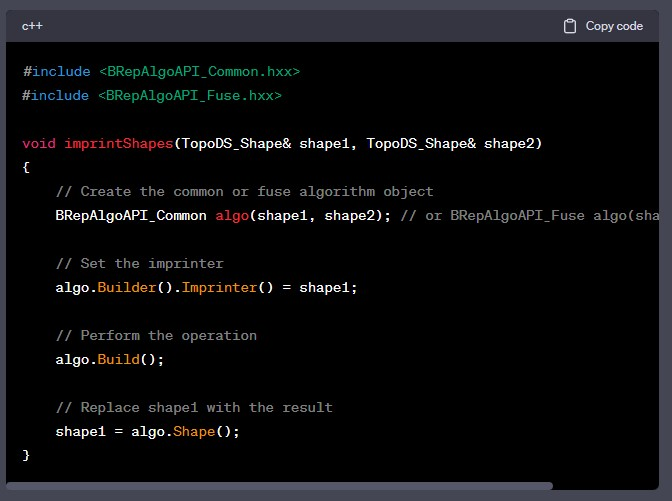The code did not compile, because the Imprinter() method does not exist. The chatbot had perhaps decided that the Imprint function was already implemented in OpenCASCADE, and so used it like other operations in this library.

Our attempt to point out the error did not help: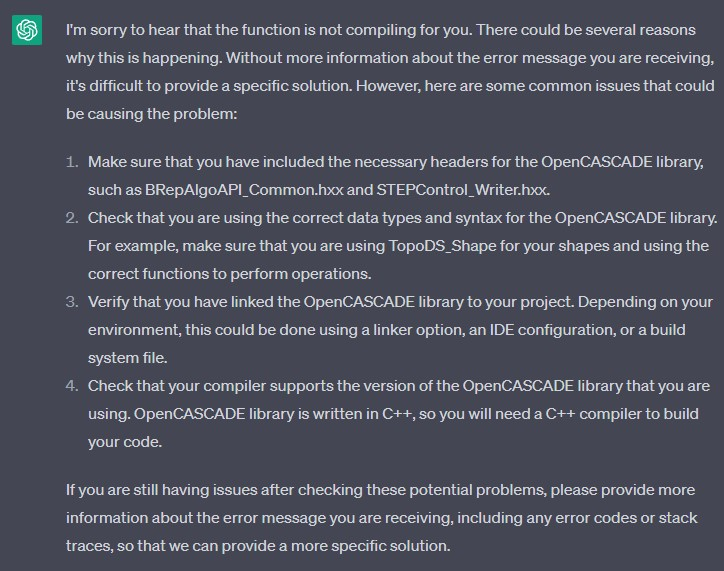We explained:And it responded with a non-existent class that implements the Imprint function:We repeated the request a few more times by adding clarifications, such as prohibiting the use of the Imprint operation, but the generated code would not compile. Here are some examples of the follow-up code: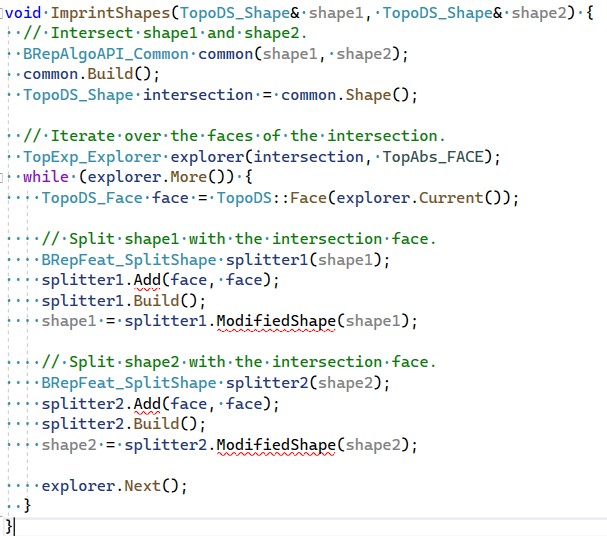As you can see, the chatbot calls not only to non-existent methods, but also misuses existing ones. The closest to a correct solution was the following code:The output is shown below. At first glance, the chatbot-generated Imprint function seems to work. The white lines on the gray faces of the cube are the edges of the intersection of the cube with the solid that was imprinted. However, the intersection lines did not subdivide the topology of the cube, which means that a full-fledged Imprint function was not implemented.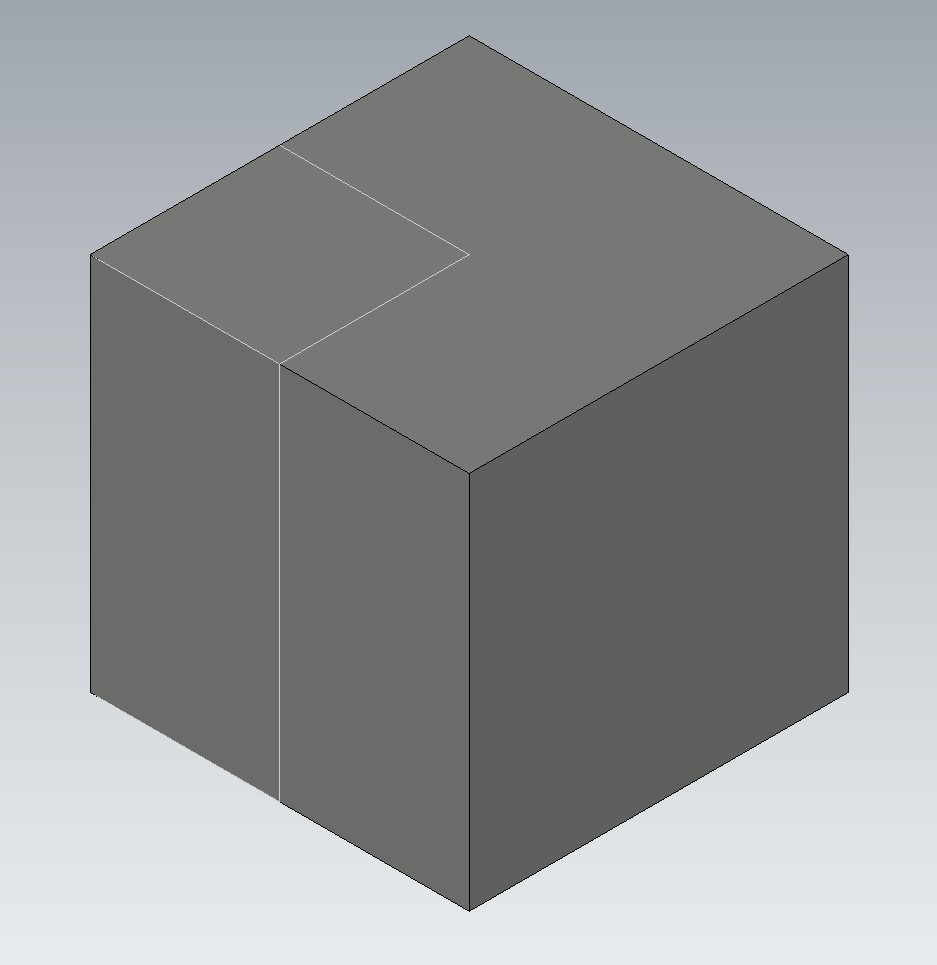We issued a dozen more requests, all of which were unsuccessful.

Summary

Based on our experience, we can summarize the following:

• ChatGPT employs imaginary OpenCASCADE API classes and methods
• ChatGPT uses non-existent class methods; it forgets to use “include”
• In some cases, ChatGPT needs to be guided as to which classes to use for implementation

### Task 3. Describe How to Implement a 3D Offset Algorithm

Our third task required no coding. Instead, we asked ChatGPT to provide a brief description of a typical algorithm implemented by developers of geometric kernels. We chose the 3D Offset algorithm.

The algorithm shifts the faces of a solid by a specified value and then creates a new smaller or larger solid, depending on the direction of the shift. The intersections of the faces and possible gaps between them must be handled properly to arrive at a valid 3D solid.

The first suggestion from ChatGPT was, of course, to use CAD software: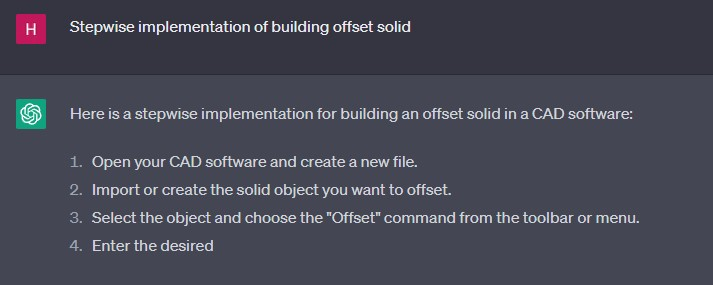But with our second attempt, the chatbot gave a correct, albeit general, explanation of how to implement 3D offsets of solids:The text looked to us like it had been copied from an article or a software manual, so we asked about the resources available to explore the topic further: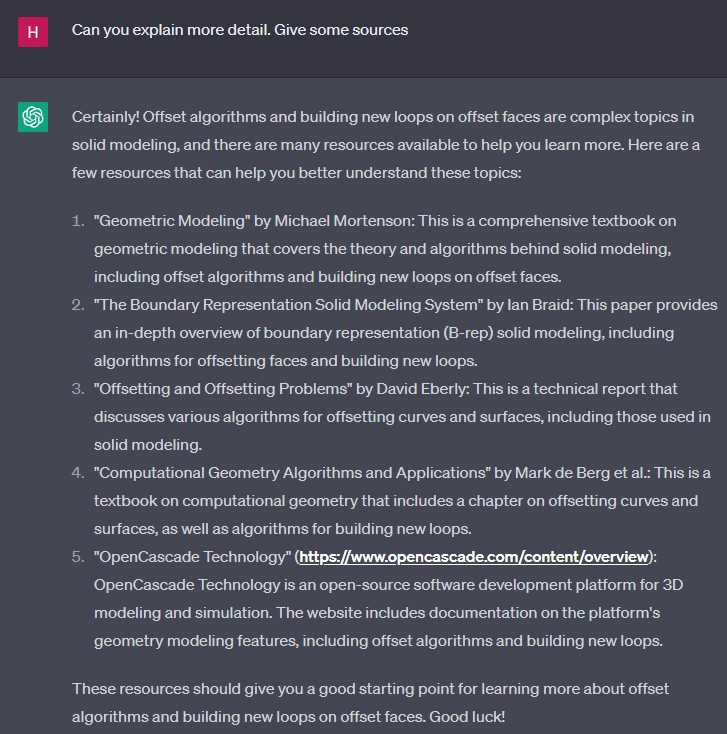The links were, unfortunately, not useful. For instance, there is no information about offsets in the Michael Mortenson book “Geometric Modeling.” The scientific articles in the list do not exist. The link to the OpenCASCADE Website does not open.

Summary

• ChatGPT can roughly explain algorithms
• ChatGPT cannot provide detailed explanations with correct references

### Conclusion

Although the chatbot did not complete all the tasks as we expected, we found it useful as an assistant that suggests ideas for solutions or for generating basic code.

The benefit of a chatbot depends on how well and how accurately one writes requests, so more ChatGPT users with more experience might obtain better results for the same tasks.

For now, the answer to the question “Can ChatGPT replace a LEDAS employee?” is definitely “No!”